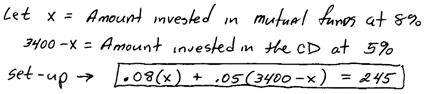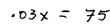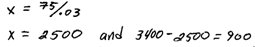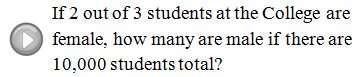## Pages

### Ratio and Proportion ApplicationsWhen setting up proportions, be sure to be consistent.  Units for the numerators should be the same and units for the denominators should be the same as well. After obtaining an equation with two equal fractions, cross multiply.

Proportion Problem: If 2 out of 3 dentists prefer Crest toothpaste, how many prefer Crest out of 600 dentists surveyed?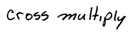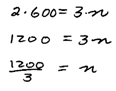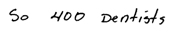Proportion Problem: In Visalia 3 out of every 7 voters said yes to proposition 40.  If 42,000 people voted, how many said no to proposition 40?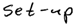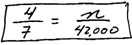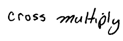Proportion Problem: A recipe calls for 5 tablespoons of sugar for every 8 cups of flour.  How many tablespoons of sugar are required for 32 cups of flour?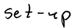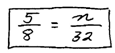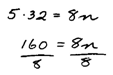Coin Problem: Sally has 12 coins consisting of quarters and dimes.  The value adds to \$2.25.  How many of each coin does she have?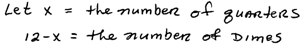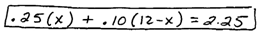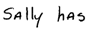Mixture Problem: A 50% alcohol solution is mixed with a 10% alcohol solution to create 8 ounces of a 32% alcohol solution.  How much of each is needed?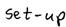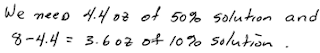Interest Problem: Mary invested her total savings of \$3,400 in two accounts.  Her mutual fund account earned 8% interest last year and her CD earned 5%.  If her total interest for the year was \$245, how much was in each account?